Next: Identifiability of the ICA Up: Independent Component Analysis Previous: Statistical independence

## Definitions of linear independent component analysis

Now we shall define the problem of independent components analysis, or ICA. We shall only consider the linear case here, though non-linear forms of ICA also exist. In the literature, at least three different basic definitions for linear ICA can be found [36,80], though the differences between the definitions are usually not emphasized. This is probably due to the fact that ICA is such a new research topic: most research has concentrated on the simplest one of these definitions. In the definitions, the observed m-dimensional random vector is denoted by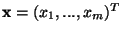.

The first and most general definition is as follows:

Definition 1 (General definition)   ICA of the random vector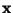consists of finding a linear transform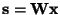so that the components si are as independent as possible, in the sense of maximizing some function F(s1,...,sm) that measures independence.

This definition is the most general in the sense that no assumptions on the data are made, which is in contrast to the definitions below. Of course, this definition is also quite vague as one must also define a measure of independence for the si. One cannot use the definition of independence in Eq. (7), because it is not possible, in general, to find a linear transformation that gives strictly independent components. The problem of defining a measure of independence will be treated in the next Section. A different approach is taken by the following more estimation-theoretically oriented definition:

Definition 2 (Noisy ICA model)   ICA of a random vectorconsists of estimating the following generative model for the data: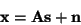(10)

where the latent variables (components) si in the vector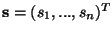are assumed independent. The matrix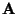is a constant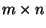'mixing' matrix, and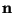is a m-dimensional random noise vector.

This definition reduces the ICA problem to ordinary estimation of a latent variable model. However, this estimation problem is not very simple, and therefore the great majority of ICA research has concentrated on the following simplified definition:

Definition 3 (Noise-free ICA model)   ICA of a random vectorconsists of estimating the following generative model for the data: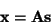(11)

whereand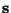are as in Definition 2.

Here the noise vector has been omitted. This is also the model introduced by Jutten and Hérault in their seminal paper  (and earlier by Jutten in his PhD  in French), which was probably the earliest explicit formulation of ICA (but see ).

In this paper, we shall concentrate on this noise-free ICA model definition. This choice can be partially justified by the fact that most of the research on ICA has also concentrated on this simple definition. Even the estimation of the noise-free model has proved to be a task difficult enough. The noise-free model may be thus considered a tractable approximation of the more realistic noisy model. The justification for this approximation is that methods using the simpler model seem to work for certain kinds of real data, as will be seen below. The estimation of the noisy ICA model is treated in Section 6.

It can be shown  that if the data does follow the generative model in Eq. (11), Definitions 1 and 3 become asymptotically equivalent, if certain measures of independence are used in Definition 1, and the natural relation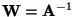is used with n=m.Next: Identifiability of the ICA Up: Independent Component Analysis Previous: Statistical independence
Aapo Hyvarinen
1999-04-23